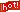5月前 北大袁萌关于数学极限定义的量词组合复杂度

在数学中 ，使用的量词只有两大类：“”与“”。

请见本文附件文章。

Comparison with infinitesimal definition

Keisler proved that a hyperreal definition of limit reduces the quantifier complexity by two quantifiers.] Namely,

f ( x )  converges to a limit L as x  tends to a if and only if for every infinitesimal e, the value

f ( x + e )  is infinitely close to L; see microcontinuity for a related definition of continuity, essentially due to Cauchy. Infinitesimal calculus textbooks based on Robinson's approach provide definitions of continuity, derivative, and integral at standard points in terms of infinitesimals. Once notions such as continuity have been thoroughly explained via the approach using microcontinuity, the epsilon–delta approach is presented as well. Karel Hrbáek argues that the definitions of continuity, derivative, and integration in Robinson-style non-standard analysis must be grounded in the ε–δ method in order to cover also non-standard values of the input. Baszczyk et al. argue that microcontinuity is useful in developing a transparent definition of uniform continuity, and characterize the criticism by Hrbáek as a "dubious lament".Hrbáek proposes an alternative non-standard analysis, which (unlike Robinson's) has many "levels" of infinitesimals, so that limits at one level can be defined in terms of infinitesimals at the next level.